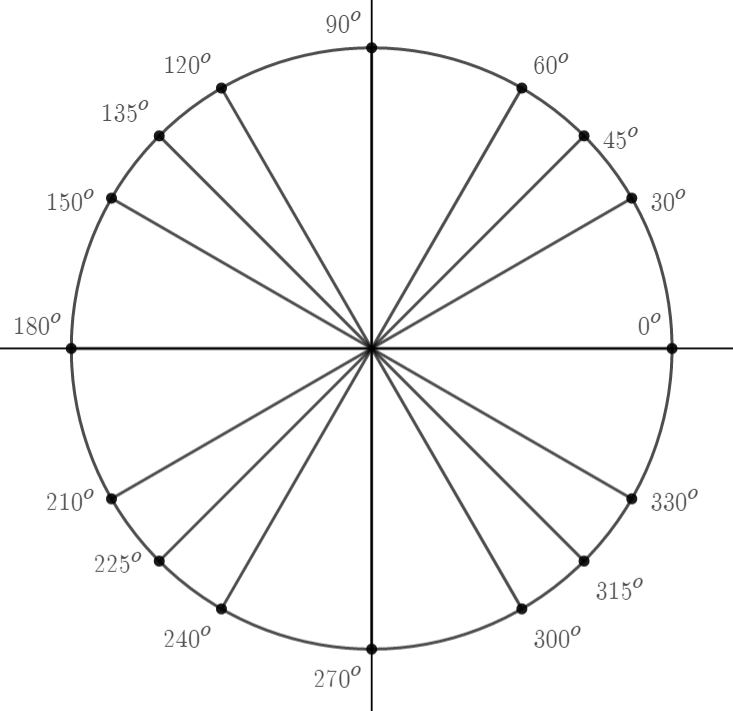# Unit Circle and Trigonometric Functions

## Objective

Find values of specific points on the unit circle using geometry.

## Common Core Standards

### Core Standards

?

• F.TF.A.2 — Explain how the unit circle in the coordinate plane enables the extension of trigonometric functions to all real numbers, interpreted as radian measures of angles traversed counterclockwise around the unit circle.

• F.TF.A.4 — Use the unit circle to explain symmetry (odd and even) and periodicity of trigonometric functions.

?

• G.SRT.D.10

• G.SRT.D.11

• G.SRT.D.9

## Criteria for Success

?

1. Identify 30-60-90 and 45-45-90 triangles in the unit circle.
2. Find coordinates of points at reference angles on the unit circle.
3. Identify reference angles on a unit circle and explain their geometric significance.

## Anchor Problems

?

### Problem 1The radius of the circle above is $1$, and it is centered at the origin. This is called a “unit circle.” Find the coordinates of the point marked at ${45^{\circ}}$.

#### Guiding Questions

Create a free account or sign in to access the Guiding Questions for this Anchor Problem.

### Problem 2The radius of the circle above is $1$, and it is centered at the origin. Find the coordinates of the point marked at $150^{\circ}$.

#### Guiding Questions

Create a free account or sign in to access the Guiding Questions for this Anchor Problem.

## Problem Set

?

The following resources include problems and activities aligned to the objective of the lesson that can be used to create your own problem set.

• Include problems determining coordinates at a variety of angles, including multiples of ${90^{\circ}}$.
• Include problems asking about the relative size of different coordinates on the unit circle without evaluating. For instance, which is larger, the $x$-value at ${34^{\circ}}$ or the $x$-value at ${35^{\circ}}$?
• Include error analysis problems where special triangles are used incorrectly.
• Include problems that require students to verify special triangles using the Pythagorean theorem.
• Include problems asking which angles on the unit circle can be found geometrically using the tools they have.

## Target Task

?Find the distance between the point labeled ${30^{\circ}}$ and the point labeled $3{30^{\circ}}$.